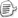### 从表达式说起

Expression Tree从名称上看就是“表达式树”的意思。许多人一看到它，就会想起Lambda表达式，委托，Linq等等一堆名词。但其实最基本的概念就是“表达式”。现在让我们把那些名词全都给忘了，来重新了解一下表达式。

 3 //常数表达式 a //变量或参数表达式 !a //一元逻辑非表达式 a + b //二元加法表达式 Math.Sin(a) //方法调用表达式 new StringBuilder() //new 表达式

### 表达式树

Expression各个子类的构造函数都是不公开的，要创建表达式树只能使用Expression类提供的静态方法。（这同时也说明表达式树体系是不能自己扩展的）如果我们要创建1 + 2 + 3这个表达式的表达式树，可以这样写：

 ConstantExpression exp1 = Expression.Constant(1); ConstantExpression exp2 = Expression.Constant(2); BinaryExpression exp12 = Expression.Add(exp1, exp2); ConstantExpression exp3 = Expression.Constant(3); BinaryExpression exp123 = Expression.Add(exp12, exp3);

### Lambda表达式

Lambda也是C#3.0/VB9新引入的表达式。我们都知道它和以前的匿名函数和委托有关。不过现在还是把这些暂时都忘掉，完全把Lambda表达式当成一种新的表达式来看到。刚才我们看到了各种各样的表达式，有的表示一个常数；有的表示一个变量；有的表示加法；有的表示函数调用等等。Lambda表达式作为一个表达式，它表达的是一个函数。Lambda表达式的成分就是一系列的参数加上一个表示函数逻辑的表达式组成。

 (parameters) => expression

Lambda表达式最重要的特色是它可以引入一批参数，这批参数可以在函数体表达式中使用。基于这种特色，我们就可以创建出带自定义变量的表达式树，而这些自定义变量就表示成Lambda表达式的参数：

 ParameterExpression expA = Expression.Parameter(typeof(double), "a"); //参数a MethodCallExpression expCall = Expression.Call(null, typeof(Math).GetMethod("Sin", BindingFlags.Static | BindingFlags.Public), expA); //Math.Sin(a) LambdaExpression exp = Expression.Lambda(expCall, expA); // a => Math.Sin(a)

 Expression> exp = a => Math.Sin(a);

• 运行时分析表达式的逻辑
• 序列化或者传输表达式
• 重新编译成可执行的代码

### 习题

-a

a + b * 2

Math.Sin(x) + Math.Cos(y)

new StringBuilder(“Hello”)

new int[] { a, b, a + b}

a[i – 1] * i

a.Length > b | b >= 0

（高难度）new System.Windows.Point() { X = Math.Sin(a), Y = Math.Cos(a) }

（待续）posted on 2009-08-28 20:01  装配脑袋  阅读(30351)  评论(88编辑  收藏  举报